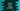# How to convert a date to ISO8601 format in python## How to convert a date to ISO8601 format in python:

ISO8601 is a way to represent date and time in an international standard. This helps to store data in a specific format and also to avoid any miscommunication while transfering data. Date-time representation is different in different countries. If everyone stick to a same format, it becomes easier for data communication.

Python provides a function to quickly convert a datetime object to ISO8601, called isoformat(). In this post, we will learn how to use isoformat() function with examples.

## isoformat Definition:

isoformat method is defined as below:

``isoformat(self, sep='T', timespec='auto')``

It returns the time in ISO8601 format. The format is ‘YYYY-MM-DD HH:MM:SS.mmmmmm’. The fractional part is removed if microseconds is equal to 0.

If timezone info is not None, the UTC value is also added to the end like ‘YYYY-MM-DD HH:MM:SS.mmmmmm+HH:MM’.

sep and timespec are optional values. sep is for changing the separator, default is T. And, timespec is the number of time terms to include in the result. It is auto by default. But we can also provide ‘hours’, ‘minutes’, ‘seconds’, ‘milliseconds’ and ‘microseconds’.

## Example of isoformat with datetime:

Let me show you an example of how isoformat works:

``````from datetime import datetime

current_datetime = datetime.now()

print(current_datetime.isoformat())``````

We are converting a datetime object to isoformat. It is the current date-time info.

If you run this program, it will print one output as like below:

``2021-07-01T23:06:36.230754``

## Converting a date object using isoformat:

We can also call isoformat() on a date object as like below:

``````from datetime import date

current_date = date(2020, 6, 12)

print(current_date.isoformat())``````

It will print:

``2020-06-12``

### Changing the separator using isoformat:

We can use the sep parameter to change the current separator, which is T by default.

``````from datetime import datetime

current_datetime = datetime.now()

print(current_datetime.isoformat(sep='X'))``````

It will print:

``2021-07-01X23:11:03.842676``

Changing the separator is not a good idea because T is the recommended separator.

### Changing timespec:

Let’s use timespec with different values:

``````from datetime import datetime

current_datetime = datetime.now()

print(current_datetime.isoformat(timespec='auto'))
print(current_datetime.isoformat(timespec='hours'))
print(current_datetime.isoformat(timespec='minutes'))
print(current_datetime.isoformat(timespec='seconds'))
print(current_datetime.isoformat(timespec='milliseconds'))
print(current_datetime.isoformat(timespec='microseconds'))``````

It will print:

``````2021-07-21T13:13:46.697389
2021-07-21T13
2021-07-21T13:13
2021-07-21T13:13:46
2021-07-21T13:13:46.697
2021-07-21T13:13:46.697389``````